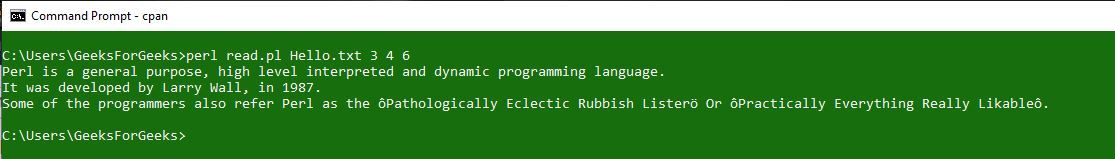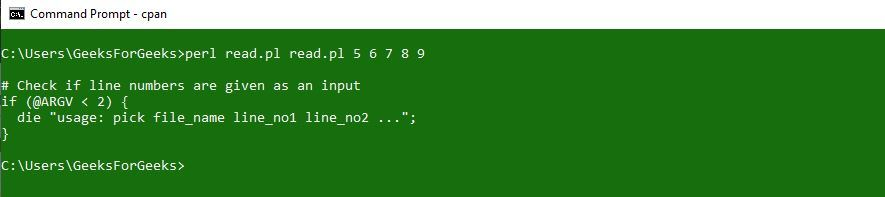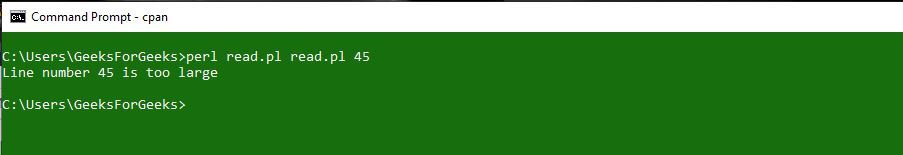Open in App
Not now

# Display file contents based on line number

• Last Updated : 28 Aug, 2019

Write a Perl program to display contents of the file in sorted order(ascending) based on line numbers given via command-line arguments. Note that the line numbers can be in any order and throw an error if the line number is large.

Syntax: perl `Filename.pl` `File_to_be_read.abc` `x y z`
Here,
Filename.pl is the name of the file that contains the perl script
File_to_be_read.abc is the name of the file which is to be read. This file can be of any type. Ex- text, script, etc.
x y z are the line numbers which are to be printed.

Approach:
Sort the line numbers excluding the first argument which is file name. Once sorted read whole content of the file into an array using single statement (my @file = <FNAME>). Now loop through the sorted line numbers and display the file content by passing line number as index to file array like (print “\$file[\$var-1]\n”;).

Example 1: Consider a file Hello.txt

 `#!/usr/bin/perl ` `use` `warnings; ` `use` `strict; ` `  `  `# Check if line numbers are given as an input ` `if` `(``@ARGV` `< 2) ` `{ ` `    ``die` `"usage: pick file_name line_no1 line_no2 ..."``; ` `} ` `  `  `open` `FNAME, ``\$ARGV`` or ``die` `"cannot open file"``; ` ` `  `# Exclude the first argument  ` `# for sorting line numbers ` `shift` `(``@ARGV``);  ` `my` `@line_numbers` `= ``sort` `{ ``\$a` `<=> ``\$b` `} ``@ARGV``;  ` `  `  `# Read whole file content into an array  ` `# and removes new line using chomp() ` `chomp` `(``my` `@file` `= ); ` `  `  `foreach` `my` `\$var` `(``@line_numbers``)  ` `{ ` `    ``if` `(``\$var``> ``\$#file``) ` `    ``{ ` `        ``print` `"Line number \$var is too large\n"``; ` `        ``next``; ` `    ``} ` `    ``print` `"\$file[\$var-1]\n"``; ` `} ` `close` `FNAME; `

Output:Example 2: Reading the same script file

 `#!/usr/bin/perl ` `use` `warnings; ` `use` `strict; ` `  `  `# Check if line numbers are given as an input ` `if` `(``@ARGV` `< 2) ` `{ ` `    ``die` `"usage: pick file_name line_no1 line_no2 ..."``; ` `} ` `  `  `open` `FNAME, ``\$ARGV`` or ``die` `"cannot open file"``; ` ` `  `# Exclude the first argument  ` `# for sorting line numbers ` `shift` `(``@ARGV``);  ` `my` `@line_numbers` `= ``sort` `{ ``\$a` `<=> ``\$b` `} ``@ARGV``;  ` `  `  `# Read whole file content into an array  ` `# and removes new line using chomp() ` `chomp` `(``my` `@file` `= ); ` `  `  `foreach` `my` `\$var` `(``@line_numbers``)  ` `{ ` `    ``if` `(``\$var``> ``\$#file``) ` `    ``{ ` `        ``print` `"Line number \$var is too large\n"``; ` `        ``next``; ` `    ``} ` `    ``print` `"\$file[\$var-1]\n"``; ` `} ` `close` `FNAME; `

Output:If the line number passed is not in the file:My Personal Notes arrow_drop_up
Related Articles